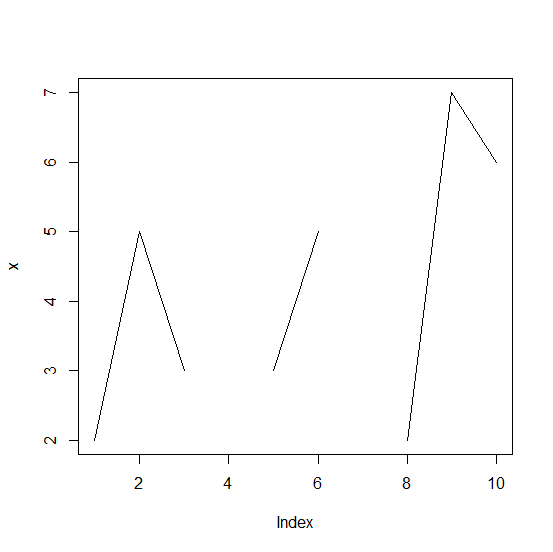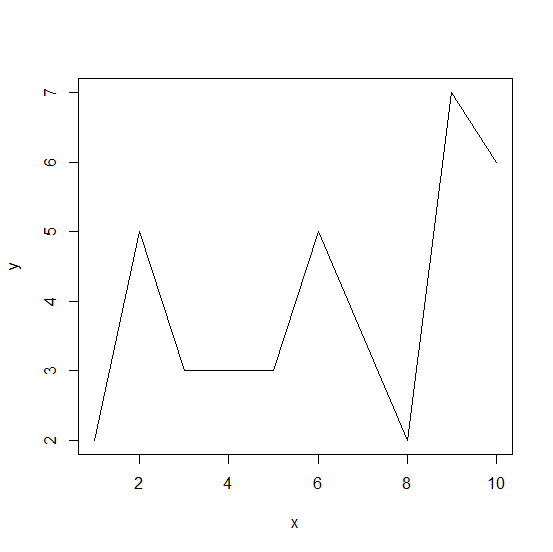# How to create the plot of a vector that contains missing values by adding the missing values in base R?

If there are missing values in a vector then the plot of such vector will not have all the values, only the non−missing values will be shown. If we want to create the plot by adding the missing values in the plot then we need to define the X−axis for the length of the vector and the Y−axis with the actual vector using cbind but missing values will be omitted as shown in the below example.

## Example

Live Demo

x<−c(2,5,3,NA,3,5,NA,2,7,6)
plot(x,type="l")

## OutputCreating the plot by adding the missing values

## Example

plot(na.omit(cbind(x=seq_along(x),y=x)),type="l")

## Output